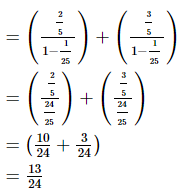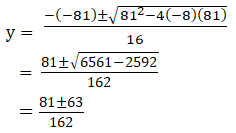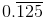• +91 9971497814
• info@interviewmaterial.com

# RD Chapter 20- Geometric Progressions Ex-20.4 Interview Questions Answers

### Related Subjects

Question 1 :

Find the sum of the following series to infinity:

(i) 1 – 1/3 + 1/32 – 1/33 + 1/34 +… ∞

(ii) 8 + 4√2 + 4 + …. ∞

(iii) 2/5 + 3/52 + 2/53 + 3/54 +…. ∞

(iv) 10 – 9 + 8.1 – 7.29 + …. ∞

(i) 1 – 1/3 + 1/32 –1/33 + 1/34 + … ∞

Given:

S = 1– 1/3 + 1/32 – 1/33 + 1/34 + …∞

Where, a = 1, r = -1/3

By using the formula,

S =a/(1 – r)

= 1 / (1 – (-1/3))

= 1/ (1 + 1/3)

= 1/ ((3+1)/3)

= 1/ (4/3)

= ¾

(ii) 8 + 4√2 + 4 + ….∞

Given:

S = 8+ 4√2 + 4 + …. ∞

Where, a = 8, r =4/4√2 = 1/√2

By using the formula,

S =a/(1 – r)

= 8 / (1 – (1/√2))

= 8 / ((√2 –1)/√2)

= 8√2 /(√2 –1)

Multiply and dividewith √2 + 1 we get,

= 8√2 /(√2 –1) × (√2 + 1)/( √2 + 1)

= 8 (2 + √2)/(2-1)

= 8 (2 + √2)

(iii) 2/5 + 3/52 +2/53 + 3/54 + …. ∞

The given terms can bewritten as,

(2/5 + 2/53 +…) + (3/52 + 3/54 + …)

(a = 2/5, r = 1/25)and (a = 3/25, r = 1/25)

By using the formula,

S =a/(1 – r)(iv) 10 – 9 + 8.1 – 7.29 +…. ∞

Given:

S = 8+ 4√2 + 4 + …. ∞

Where, a = 10, r =-9/10

By using the formula,

S =a/(1 – r)

= 10 / (1 – (-9/10))

= 10 / (1 + 9/10)

= 10 / ((10+9)/10)

= 10 / (19/10)

= 100/19

= 5.263

Question 2 :

Prove that :
(91/3 . 91/9 . 91/27 ….∞) = 3.

Let us consider theLHS

(91/3 .91/9 . 91/27 ….∞)

This can be written as

91/3 + 1/9 + 1/27+ …∞

So let us consider m =1/3 + 1/9 + 1/27 + … ∞

Where, a = 1/3, r =(1/9) / (1/3) = 1/3

By using the formula,

S =a/(1 – r)

= (1/3) / (1 – (1/3))

= (1/3) / ((3-1)/3)

= (1/3) / (2/3)

= ½

So, 9m =91/2 = 3 = RHS

Hence proved.

Question 3 :

Prove that :

(21/4 .41/8 . 81/16. 161/32….∞)= 2.

Let us consider theLHS

(21/4 .41/8 .81/16. 161/32….∞)

This can be written as

21/4 .22/8 . 23/16 . 21/8 … ∞

Now,

21/4 + 2/8 + 3/16+ 1/8 + …∞

So let us consider 2x,where x = ¼ + 2/8 + 3/16 + 1/8 + … ∞ …. (1)

Multiply both sides ofthe equation with 1/2, we get

x/2 = ½ (¼ + 2/8 +3/16 + 1/8 + … ∞)

= 1/8 + 2/16 + 3/32 +… + ∞ …. (2)

Now, subtract (2) from(1) we get,

x – x/2 = (¼ + 2/8 +3/16 + 1/8 + … ∞) – (1/8 + 2/16 + 3/32 + … + ∞)

By grouping similarterms,

x/2 = ¼ + (2/8 – 1/8)+ (3/16 – 2/16) + … ∞

x/2 = ¼ + 1/8 + 1/16 +… ∞

x = ½ + ¼ + 1/8 + 1/16+ … ∞

Where, a = 1/2, r =(1/4) / (1/2) = 1/2

By using the formula,

S =a/(1 – r)

= (1/2) / (1 – 1/2)

= (1/2) / ((2-1)/2)

= (1/2) / (1/2)

= 1

From equation (1), 2x =21 = 2 = RHS

Hence proved.

Question 4 :

If Sp denotes the sum of the series 1 + rp +r2p + … to ∞ and sp the sum of the series 1 – rp +r2p – … to ∞, prove that sp + Sp =2 S2p.

Given:

Sp = 1+ rp + r2p + … ∞

By using the formula,

S =a/(1 – r)

Where, a = 1, r = rp

So,

Sp = 1/ (1 – rp)

Similarly, sp =1 – rp + r2p – … ∞

By using the formula,

S =a/(1 – r)

Where, a = 1, r = -rp

So,

Sp = 1/ (1 – (-rp))

= 1 / (1 + rp)

Now, Sp +sp = [1 / (1 – rp)] + [1 / (1 + rp)]

2S2p =[(1 – rp) + (1 + rp)] / (1 – r2p)

= 2 /(1 – r2p)

2S2p =Sp + Sp

Question 5 : Find the sum of the terms of an infinite decreasing G.P. in which all the terms are positive, the first term is 4, and the difference between the third and fifth term is equal to 32/81.

Let ‘a’ be the firstterm of GP and ‘r’ be the common ratio.

We know that nth termof a GP is given by-

an =arn-1

As, a = 4 (given)

And a5 –a3 = 32/81 (given)

4r4 –4r2 = 32/81

4r2(r2 –1) = 32/81

r2(r2 –1) = 8/81

Let us denote r2 withy

81y(y-1) = 8

81y2 –81y – 8 = 0

Using the formula ofthe quadratic equation to solve the equation, we gety = 18/162 = 1/9 or

y = 144/162

= 8/9

So, r2 =1/9 or 8/9

= 1/3 or 2√2/3

We know that,

Sum of infinite, S =a/(1 – r)

Where, a = 4, r = 1/3

S = 4/ (1 – (1/3))

= 4 / ((3-1)/3)

= 4 / (2/3)

= 12/2

= 6

Sum of infinite, S =a/(1 – r)

Where, a = 4, r = 2√2/3

S = 4/ (1 – (2√2/3))

= 12 / (3 – 2√2)

Question 6 : Express the recurring decimal 0.125125125 … as a rational number.

Given:

0.125125125

So, 0.125125125 == 0.125 + 0.000125 +0.000000125 + …

This can be written as

125/103 +125/106 + 125/109 + …

125/103 [1+ 1/103 + 1/106 + …]

By using the formula,

S =a/(1 – r)

125/103 [1/ (1 – 1/1000)]

125/103 [1/ ((1000 – 1)/1000))]

125/103 [1/ (999/1000)]

125/1000 (1000/999)

125/999

The decimal0.125125125 can be expressed in rational number as 125/999

Todays Deals### RD Chapter 20- Geometric Progressions Ex-20.4 Contributorskrishan

Name:
Email:

# Latest News# 9000 interview questions in different categories